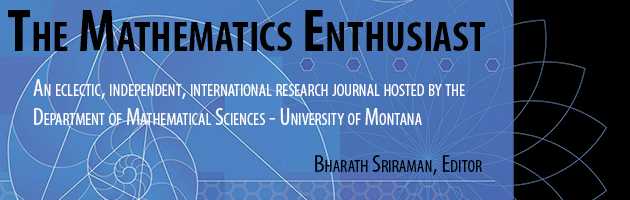•
•### Understanding Point and Slope in Linear Equations and Approximations: A Case Study

21

3

#### Abstract

This article delves into an intervention designed to enhance a precalculus student’s understanding in constructing linear equations and in approximating function values. Before the intervention, the student primarily relied on the algebraic formula y2-y1/x2-x1 to determine slope, knew slope geometrically as Δyx solely for integer values, and struggled to construct a linear equation without an explicitly shown y-intercept. Through the intervention, the student comprehended slope m as a unit rate and expanded this understanding to Δy = 𝑚Δ𝑥 for integer Δx by iterating m, Δ𝑥 number of times. She also successfully used this newfound understanding to construct linear equations, including tangent line equations, and approximate function values. Nonetheless, she faced some challenges in comprehending Δy = 𝑚Δ𝑥 for non-integer Δ𝑥 and in conceiving point as a multiplicative object with its coordinates representing covarying quantities. Further exploration is needed to validate these findings and address potential difficulties that students might face in grasping slope in the context of linear equations and approximations.

541

568

#### Digital Object Identifier (DOI)

10.54870/1551-3440.1642

#### Publisher

University of Montana, Maureen and Mike Mansfield Library

COinS